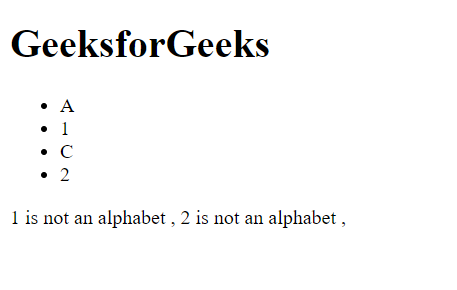# HTML DOM matches() Method

• Last Updated : 29 Jun, 2020

The matches() method checks if the Element that would be selected by the provided selectorString is the selector. If the specified element is the valid selector then it will return true, else false.

Syntax:

`var boolean_result = element.matches("selector");`

Parameters: Here, selector is used as a parameter.

Return value: It returns Boolean value i.e. (True when selector matches else false).

Example: In this example, there is a list in which there are some elements that have class selector as number. So, we stored the list in a variable and by iterating on the list, if selector matches number class, then printing on the document correspondingly.

## HTML

 ```<``html``>`` ` `<``head``>``    ``<``title``>``        ``HTML DOM matches() Method``    `````` ` `<``body``>``    ``<``h1``>GeeksforGeeks`` ` `    ``<``ul` `id``=``"abc"``>``        ``<``li``>A``        ``<``li` `class``=``"number"``>1``        ``<``li``>C``        ``<``li` `class``=``"number"``>2``    ```` ` `    ``<``script``>``        ``var abc = document.getElementsByTagName('li');``        ``for (var i = 0; i < ``abc.length``; i++) {``            ``if (abc[i].matches('.number')) {``                ``document.write(abc[i].textContent ``                    ``+ ' is not an alphabet ,  ');``            ``}``        ``}``    `````` ` ``

Output:Note: It is supported by all the browsers.

My Personal Notes arrow_drop_up